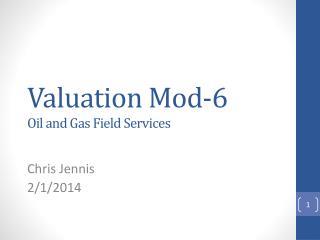Download PresentationValuation Mod-6 Oil and Gas Field Services

# Valuation Mod-6 Oil and Gas Field Services

Download Presentation## Valuation Mod-6 Oil and Gas Field Services

- - - - - - - - - - - - - - - - - - - - - - - - - - - E N D - - - - - - - - - - - - - - - - - - - - - - - - - - -
##### Presentation Transcript

1. Valuation Mod-6Oil and Gas Field Services Chris Jennis 2/1/2014

2. Agenda Module 6 Summary Cost of Equity Cost of Debt WACC New DCF Questions

3. Module 6 • “Improving the cost of capital assumption” • Cost of Equity • Use of CAPM • Cost of Debt Capital • Market interest rate on debt instruments of the firm • Borrowing rate depends on perceived level of risk in eyes of the lenders • AAA are very unlikely to default • BBB- and higher are investment grade • Cost of Capital from Cost of Debt and Cost of Equity • Weighted Average

4. Cost of Equity

5. Cost of Equity • Equation Inputs • Beta- sensitivity of the asset’s market return to overall market return • Firm Specific • Market Beta= 1 • Risk Free Rate- taken from long-term U.S. treasury bills • Return on Market • Market Risk Premium

6. Cost of Equity • Estimating Schlumberger’s Inputs • Beta- Stock’s covariance with market returns • Monthly Returns from Yahoo Finance for SLB and S&P 500 • Run Regression with S&P 500 as independent variable and SLB as dependent variable

7. Cost of Equity Data

8. Cost of Equity Regression Using Returns-Risk Free

9. Cost of Equity Regression without Risk Free Rate (Bloomberg Version)

10. Cost of Equity Outside Betas

11. Cost of Equity • My Choice of Beta • Outside sources: Ranges from 1.13-1.75 • Internal sources: .88 or 1.52 • Average of outside: 1.44 • Most comfortable with 1.52

12. Cost of Equity • Risk Free Rate and Market Rate • Risk Free Rate • Yahoo Finance • r(rf) = 3.687% • Market Rate • Goldman Sachs • ~4.5%

13. Cost of Equity Final Cost of Equity • r(rf) = 3.687% • Beta = 1.52 • r(mkt) – r(rf) = 4.5% • r(Eq)= 10.52%

14. Cost of Debt Capital

15. Cost of Debt Capital • 2 Methods • Formulaic Method • (Interest Expense/Average amount of Interest Bearing Debt) * (1-T) • T= 37% • FEAT/NFL • F/S Disclosure Method • Average L-T borrowing rate * (1-T)

16. Cost of Debt Capital • Formulaic Method

17. Cost of Debt Capital • F/S Disclosure Method • “The weighted average interest rate on variable rate debt as of December 31, 2012 was 3.6%” • Borrowing Rate= 3.6% • Tax Rate = 37% • r(debt) = 2.268%

18. Cost of Capital: WACC

19. Cost of Capital from Cost of Equity and Debt • With Formula Cost of Debt • r(debt)= 5% • V(debt)= \$ 11,190 • V(Eq)= \$117,245 • V(Ent)= \$ 128,435 • r(equity)= 10.52% • WACC= 10.04% • With F/S Disclosure Cost of Debt • r(debt)= 2.27% • V(debt)= \$ 11,190 • V(Eq)= \$117,245 • V(Ent)= \$ 128,435 • r(equity)= 10.52% • WACC= 9.80%

20. Discounted Cash Flow

21. Discounted Cash Flow Model

22. Questions?